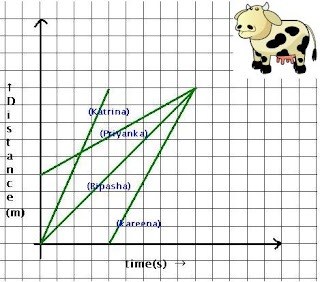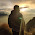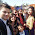## Saturday, 15 December 2012

### CBSE Class 9 - PSA Quiz (Physics)

PSA Quiz (Physics)
(Passage based MCQs)
The distance time graph shows the motion of four cows walking from the grass land to their sheds. Choose the appropriate option from multiple choices given:

Q1: Based on average velocity, which cow is the fastest?

(a) Kareena
(b) Katrina
(c) Priyanka
(d) Bipasha

Q2: Based on average velocity, which cow is the slowest?

(a) Kareena
(b) Katrina
(c) Priyanka
(d) Bipasha

Q3: Slope of distance-time graph gives information about

(a) total distance
(b) speed
(c) total displacement
(d) acceleration

Q4: Which cow starts very late?

(a) Kareena
(b) Katrina
(c) Priyanka
(d) Bipasha

Q5: Which cow covers the least distance?

(a) Kareena
(b) Katrina
(c) Priyanka
(d) Bipasha

(Standalone MCQs)

Q6: What is the SI unit of energy?

(a)  Newton
(b)  Joule
(c)  Erg
(d)  Watt

Q7: A ball is thrown upwards vertically. What is the velocity of the ball at its highest point?

(Take g = 9.8 m/s2)

(a) 9.8 m/s
(b) 1 m/s
(c) 0 m/s
(d) cannot be determined since time is not given.

Q8: An object of mass 20 kg moves with uniform acceleration from rest to a speed of 2m/s in 20 secs. On average how much power is generated in 20 seconds duration.

(a) 10 W
(b) 20 W
(c) 40 W
(d) 2 W

Q9(NSEJS 2012): Wavelength is
(i) The distance traveled by the wave in one period of oscillation of particles in the medium.
(ii) The distance between two particles, which are in the same phase.
(iii) Half of the distance between two particles, which are in the same phase.
The correct definitions are

(a) (i) and (iii)
(b) (i) and (ii)
(c) (i), (ii) and (iii)
(d) (ii) and (iii)

Q10: If we increase the time period of a wave, its frequency will

(a) decrease
(b) increase
(c) remains same
(d) none of these

Average speeds are:
katrina = 9units/4units   (fastest)
priyanka = 5/9   (slowest)
bipasha = 9/9
karrena = 9/5

1: (b) Katrina
2: (c) Priyanka
3: (b) speed
4: (a) Kareena
5: (c) Priyanka
6: (b)  Joule
7: (c) 0 m/s
8: (d) 2 W (Work done = Change in Kinetic energy = ½ × 20 × 4 = 40J, Power = 40H/20s = 2W)
9: (b) (i) and (ii)
10: (a) decrease1.sir this site is the very informative for me
Click here For Get latest Govt Jobs news and result,quiz
thank you

2.help full

helping

3.its best site ever to solve my school homework and to prepare for exams it also helped me to prepare for olympiads and international talent hunt olympiad thats why am saying its best

4.not helpful it is very easy